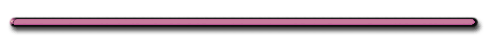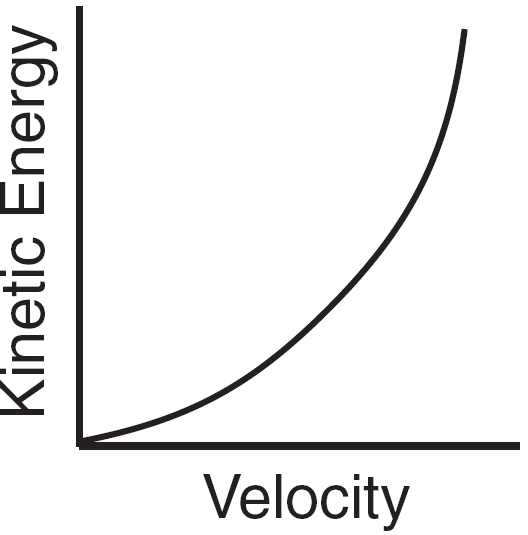HomeWhat causes the pressure in a gas?B) Kinetic Energy - the energy an object possesses due to its' motionEquation

 KE = (½)mv2Work/KE

W = Fd = ∆KE

F = average Force

W neg : decrease KE

W + : increase KE

Ex) A softball having a mass of .25 kg is pitched at 95 km/hr. By the time it reaches the plate the ball slowed to by 10%.

Neglecting gravity, estimate the average force of friction if the distance to the plate = 15 m

W = Fd = ∆KE

Convert to m/s

95 km/hr [1000m/1km][1 hr/3600 sec]

= 26.39 m/s = V1

slowed to by 10%

.9[26.39 m/s] = 23.75 m/s = V2

∆KE = 1/2m[V22 - V12 ]

Fd = ∆KE

F = ∆KE/d

F = -1.1 N

p. 162) 20Ex) baseball (m = 140 g)  travelling 32 m/s caught by a glove and moves glove back 25 cm.

Average F of ball on glove?

Work of glove on ball = ΔKE

Wball = Fd = ∆KE

Fd = 1/2m[V22 - V12 ]

F[.25m] = 1/2[.14kg][0 - (32m/s)2 ]

Fball[.25m] = -72 J

Fball =  -2.9 x 102 N

Average F of ball on glove?

Fglove =  2.9 x 102 NPower